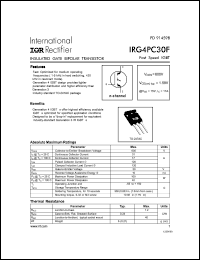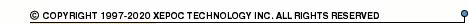More than479 017 registered clientsIRG4PC30 series datasheets. Manufacturer: International Rectifier.

 IRG4PC30 Insulated gate bipolar transistor. VCES = 600V, VCE(on)typ. = 1.59V @ VGE = 15V, IC = 17A in 3-pin TO-247AC package. Operational temperature range from -55°C to 150°C. Datasheet*) IRG4PC30FD Insulated gate bipolar transistor with ultrafast soft recovery diode. VCES = 600V, VCE(on)typ. = 1.59V @ VGE = 15V, IC = 17A in 3-pin TO-247AC package. Operational temperature range from -55°C to 150°C. Datasheet*) IRG4PC30K Insulated gate bipolar transistor. VCES = 600V, VCE(on)typ. = 2.21V @ VGE = 15V, IC = 16A in 3-pin TO-247AC package. Operational temperature range from -55°C to 150°C. Datasheet*) IRG4PC30KD Insulated gate bipolar transistor with ultrafast soft recovery diode. VCES = 600V, VCE(on)typ. = 2.21V @ VGE = 15V, IC = 16A in 3-pin TO-247AC package. Operational temperature range from -55°C to 150°C. Datasheet*) IRG4PC30S Insulated gate bipolar transistor. VCES = 600V, VCE(on)typ. = 1.4V @ VGE = 15V, IC = 18A in 3-pin TO-247AC package. Operational temperature range from -55°C to 150°C. Datasheet*) IRG4PC30U Insulated gate bipolar transistor. VCES = 600V, VCE(on)typ. = 1.95V @ VGE = 15V, IC = 12A in 3-pin TO-247AC package. Operational temperature range from -55°C to 150°C. Datasheet*) IRG4PC30UD Insulated gate bipolar transistor with ultrafast soft recovery diode. VCES = 600V, VCE(on)typ. = 1.95V @ VGE = 15V, IC = 12A in 3-pin TO-247AC package. Operational temperature range from -55°C to 150°C. Datasheet*) IRG4PC30W Insulated gate bipolar transistor. VCES = 600V, VCE(on)typ. = 2.70V @ VGE = 15V, IC = 12A in 3-pin TO-247AC package. Operational temperature range from -55°C to 150°C. Datasheet*) IRG4PC30F INSULATED GATE BIPOLAR TRANSISTOR(Vces=600V, Vce(on)typ.=1.59V, @Vge=15V, Ic=17A) Datasheet*)